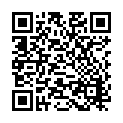Lavoisier S.A.S.
14 rue de Provigny
94236 Cachan cedex
FRANCE

Heures d'ouverture 08h30-12h30/13h30-17h30
Tél.: +33 (0)1 47 40 67 00
Fax: +33 (0)1 47 40 67 02Url courte ou permalien : www.lavoisier.fr/livre/notice.asp?ouvrage=1275276

# Mathematical logic undergraduate texts in mathematics (2nd ed 1994 corr 2nd printing) (2nd Ed., 2nd ed. 1994. Softcover reprint of the original 2nd ed. 1994)Undergraduate Texts in Mathematics Series

Langue : Anglais

## Auteurs : Ebbinghaus H.-D., Flum J., Thomas Wolfgang• ## Commentaire

This junior/senior level text is devoted to a study of first-order logic and its role in the foundations of mathematics: What is a proof? How can a proof be justified? To what extent can a proof be made a purely mechanical procedure? How much faith can we have in a proof that is so complex that no one can follow it through in a lifetime? The first substantial answers to these questions have only been obtained in this century. The most striking results are contained in Goedel's work: First, it is possible to give a simple set of rules that suffice to carry out all mathematical proofs, but, second, these rules are necessarily incomplete - it is impossible, for example, to prove all true statements of arithmetic. The book begins with an introduction to first-order logic, Goedel's theorem, and model theory. A second part covers extensions of first-order logic and limitations of the formal methods. The book covers several advanced topics, not commonly treated in introductory texts, such as Trachtenbrot's undecidability theorem. Fraissé's elementary equivalence, and Lindstroem's theorem on the maximality of first-order logic.
Preface, Part A: 1. Introduction, 2. Syntax of First-Order Languages, 3. Semantics of first-Order Languages, 4. A Sequent Calculus, 5. The Completeness Theorem, 6. The Lowenheim-Skolem and the Compactness Theorem, 7. The Scope of First-Order Logic, 8. Syntactic Interpretations and Normal Forms, Part B: 9. Extensions of First-Order Logic, 10. Limitations of the Formal Method, 11. Free Models and Logic Programming, 12. An Algebraic Characterization of Elementary Equivalence, 13. Lindstroem's Theorems, References, Symbol Index, Subject Index
This junior/senior level text starts with a thorough treatment of first-order logic and its role in the foundations of mathematics. It covers several advanced topics, not commonly treated in introductory texts, such as Trachtenbrot's undecidability theorem, FraÃ¯ssÃ©'s characterization of elementary equivalence, LindstrÃ¶m's theorem on the maximality of first-order logic, and the fundamentals of logic programming.

Date de parution :

15.5x23.5 cm

Sous réserve de disponibilité chez l'éditeur.

Prix indicatif 63,25 €

### Mots-clés :

#### Ces ouvrages sont susceptibles de vous intéresser

En continuant à naviguer, vous autorisez Lavoisier à déposer des cookies à des fins de mesure d'audience. Pour en savoir plus et paramétrer les cookies, rendez-vous sur la page Confidentialité & Sécurité.
FERMER